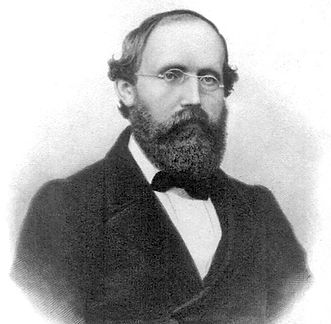# Separating the Sin and Cos PortionsThe task now is to determine when the positive portions of the even and odd sums cancel the negative portions, for both the real and imaginary sums.

The even sums can be further split into 2 sums and 2 constants using sine and cosine angle addition. Those sums and constants are given labels. At this point, it turns out that there are relationships between these new sums and constants and the previous sums.